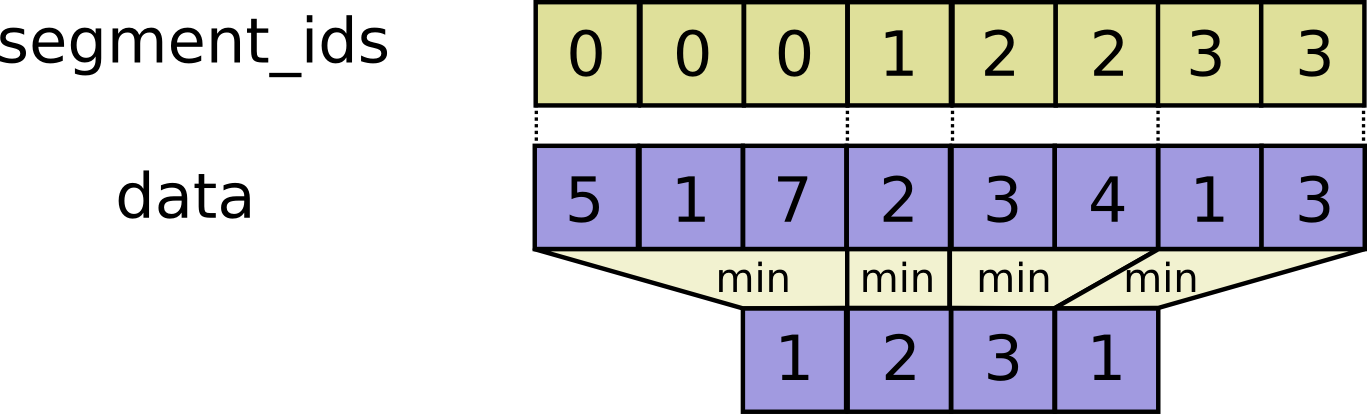Missed TensorFlow Dev Summit? Check out the video playlist.

# tf.math.segment_min

Computes the minimum along segments of a tensor.

tf.math.segment_min(
data, segment_ids, name=None
)


Read the section on segmentation for an explanation of segments.

Computes a tensor such that $$output_i = \min_j(data_j)$$ where min is over j such that segment_ids[j] == i.

If the min is empty for a given segment ID i, output[i] = 0.#### For example:

c = tf.constant([[1,2,3,4], [4, 3, 2, 1], [5,6,7,8]])
tf.segment_min(c, tf.constant([0, 0, 1]))
# ==> [[1, 2, 2, 1],
#      [5, 6, 7, 8]]


#### Args:

• data: A Tensor. Must be one of the following types: float32, float64, int32, uint8, int16, int8, int64, bfloat16, uint16, half, uint32, uint64.
• segment_ids: A Tensor. Must be one of the following types: int32, int64. A 1-D tensor whose size is equal to the size of data's first dimension. Values should be sorted and can be repeated.
• name: A name for the operation (optional).

#### Returns:

A Tensor. Has the same type as data.